﻿ Four fundamental forces and de Broglie waves

# Four fundamental forces, Hall effect, radius.

## Coulomb force is due to "unreal" virtual photons !?

### [ Quantum mechanics depends on fictitious virtual photons disobeying relativity ! ]

(Fig.1)   "Photon" is just abstract math symbol (= a ), without physical shape.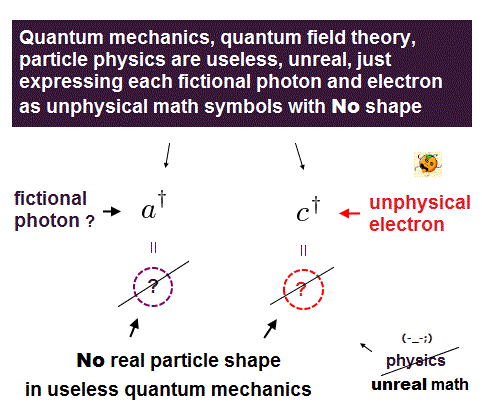Quantum mechanics claims that the classical Coulomb force is caused by photon exchange.
But clear mechanisms of "attractive" and "repulsive" Coulomb forces remain unknown.

In fact, this photon causing Coulomb force is virtual photon, so NOT real ( see this, this, this ).
Surprisingly, this virtual photon is superluminal tachyon, violating Einstein's relativity ( see this, this ).

By the way, what is the physical shape and size of a single photon ?
Unfortunately, quantum mechanics cannot answer this basic question.

In QED, these photons must be described using abstract Feynman diagram.
This Feynman QED has NO ability to show photon's clear picture at all.

It just shows meaningless math symbols ( see this, this ).
For example, a photon is created (= a ), or annihilated (= a ). That's all, NOT physics.

## "Fractional" charge quark cannot be observed !

### [ Fractional charge quark can NEVER be isolated. ← unreal quarks ! ]

(Fig.2)  The sum of quark's charge becomes integer (= +e ) !? ← Too good to be true !It is said that the proton is composed of three quarks with fractional charges.
But this fractional charges ( +2/3e, -1/3e ) can NEVER be isolated, so quark has NO real evidence.  See this and this.

They just jumped to a conclusion that Jets (= only electrons and lights can be detected ) may be quark or gluon. In standard model, quarks are just meaningless math symbols with NO physical shapes.

Originally, fractional charges such as +2/3 (= up ) and -1/3 (= down ) quarks are completely independent from (= NO relation with ) electrons.  ( Why 3/4e, 4/7e is impossible ? )

But the sum of them (= 2 × up + 1 × down quarks ) becomes just +e charge proton, which number is "accidentally" just the Same as -e charge electron in the universe !  ← Too good to be true!

On the other hand, the number of positron, which is said to be the partner of electron, is much smaller than electrons, and unstable antiparticles disappear instantly (= within 10-12 seconds ! ).

And these antiparticles disobey basic physical law such as total momenum conservation.
So standard model based on these quarks lacks reality from the beginning.

## Weak force boson disobeys energy conservation !

### [ Weinberg's weak boson inside accelerators are "different" from real β decay ! ]

(Fig.3)   In usual β decay, W boson of "80 × proton" mass cannot be found !Standard model argues, in ordinary β decay, a neutron splits into a proton and very heavy W boson.
But the mass of this W boson is 80 × protons, violating energy conservation. See this, this.

Higgs theory insists only Higgs (= 125 GeV ) can give mass to W boson, when it is produced.
The lifetimes of Higgs and W boson are very short ( ~10-22 sec ), so they easily decay.

When we get some radioactive nuclides, β decay can be seen even in ordinary laboratory without gigantic accelerators (= LHC ).

But it's impossible to detect W and Higgs boson decay (= 200 × protons energy per neutron ! ) in ordinary laboratory, meaning W and Higgs inside LHC have nothing to do with β decay, so unreal.

## Neutron is made of a proton and electron, NOT unreal quarks !

### [ Neutron is just a transient form of a proton and an electron. Quark model is unnatural. ]

(Fig.4)  Neutron is made of three "fractional" charges ? ← NOT confirmed !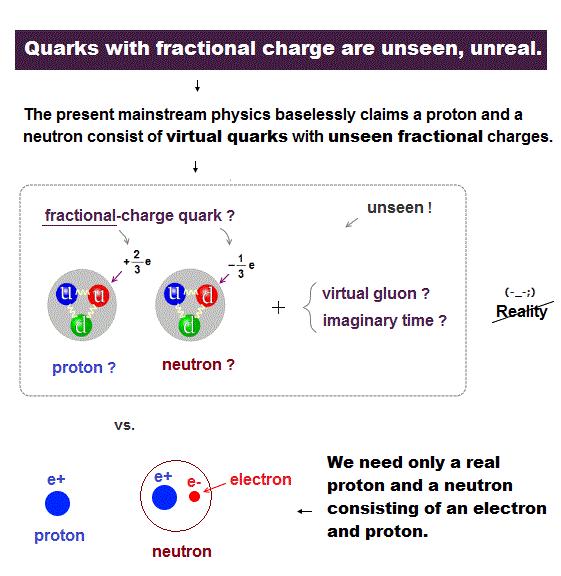Though the current particle physics claims that a neutron is made of three " fractional" charge quarks.
But these fractional charges (= 1/3e ) have never been observed.  Just speculation.

It is known that a neutron easily decays into a pair of proton and electron in 15 minutes.
So the total charge is conserved.  Neutral neutron = plus proton + minus electron.

From this fact, it is quite natural to think a neutron is made of a proton and an electron !
Because quarks of fractional charge can NEVER been isolated, so they lack experimental proofs, forever.

## Electron is stable, when its orbit is an integer times de Broglie wavelength.

### [ An integer times de Broglie wavelength prevents an electron from falling into nucleus. ]

(Fig.5)  An integer times de Broglie wavelength ← Wave ends fit each other, stable.As seen in Davisson-Germer experiment, each electron has wave-nature, and their destructive interference affects the electron's motions.

It is known that Bohr model gives exact energy levels, when its orbit satisfies an integer times de Broglie wavelength.  "Integer" means their wave ends fit each other, avoiding destructive interference.

Tedious explanations "Bohr model's accelerating electron radiates energy. " are based on completely wrong assumptions.  Because there are NO energies around only a single electron !

On this page, we can show the true mechanism of emission of electromagnetic waves and fields.

## Neutron decays = NOT-integer de Broglie wavelength.

### [ Coulomb attraction is strong. But de Broglie waves inside neutron is unstable. ]

(Fig.6)  Why does a neutron decay into proton and electron in 15 minutes ?Considering a neutron easily decays into a proton and an electron, we naturally think a neutron is some transient form of a pair of proton and electron.

As I said, each electron's motion is stable, when its orbit satisfies an integer times de Broglie wavelength, which is the reason neutron easily decays into a hydrogen atom ( n → e+, e- ).

But short distance between a proton and an electron inside a neutron generates strong Coulomb attraction, which is thought to postpone this neutron decay. It takes as much as 15 minutes.

## Proton → neutron + positron ?  neutron → Proton + .. !

### [ Though neutron decays into proton and electron, why a proton can decay into neutron ? ]

(Fig.7)  Neutron → proton + e-,  Proton → neutron +e+ ?  Perpetual machine ?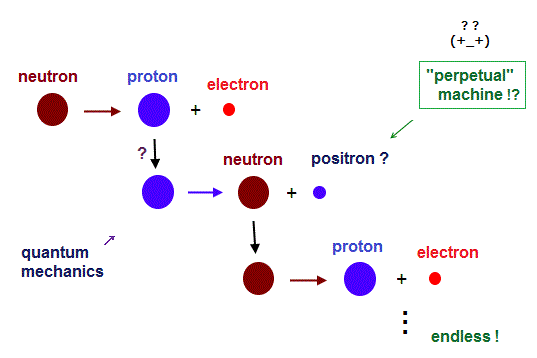Neutron is slightly heavier than a proton, so it can decay into a proton and an electron.
Surprisingly, quantum mechanics claims a proton can also decay into a neutron and a positron !

A proton is lighter than a neutron, so this inverse process is unreasonable.
If so, resultant neutron decays into proton, again, and that proton decays into a neutron, again ...

This is like a "fantasy" perpetual machine.
So the beta plus decay emitting positron violates energy conservation.

Electron capture is what actually happens in positron emission.   Unstable positron itself cannot be confirmed directly.  It is estimated only from the detection of high energy light.

## Strong force is "short-distance" Coulomb, NOT by unreal quark.

### [ An electron between two protons exerts strong attraction inside nuclei. ]

(Fig.8)  Strong nuclear force is due to short-distance Coulomb attraction !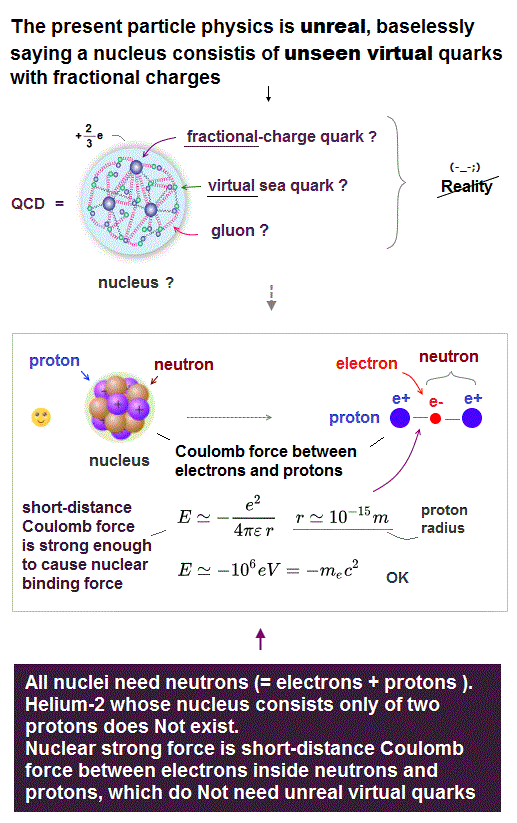Standard model claims that strong nuclear force binding positive protons inside nuclei is caused by quarks and gluon.  But neither quarks nor gluons can be isolated, so these are only hypothetical.

It is known that all nuclei are composed of protons and neutrons.
The point is, neutrons (= proton + electron ) are indispensable for making each nucleus.

A electron inside neutron is binding protons by a very "short-distance" Coulomb force.
When an electron is between two protons, total Coulomb potential energy becomes negative, and stable.

Inside tiny nucleus, these distances (= r ) are extremely short, so Coulomb attraction is close to infinity ( theoretically ), which can explain strong nuclear force, NOT depending on "fantasy" quark !

## Electrons between protons exert strong attraction in nuclei !

### [ Electron is oscillating and binding protons in nuclei ! ]

(Fig.9)  Nuclear structures in Deuterium and Helium-4.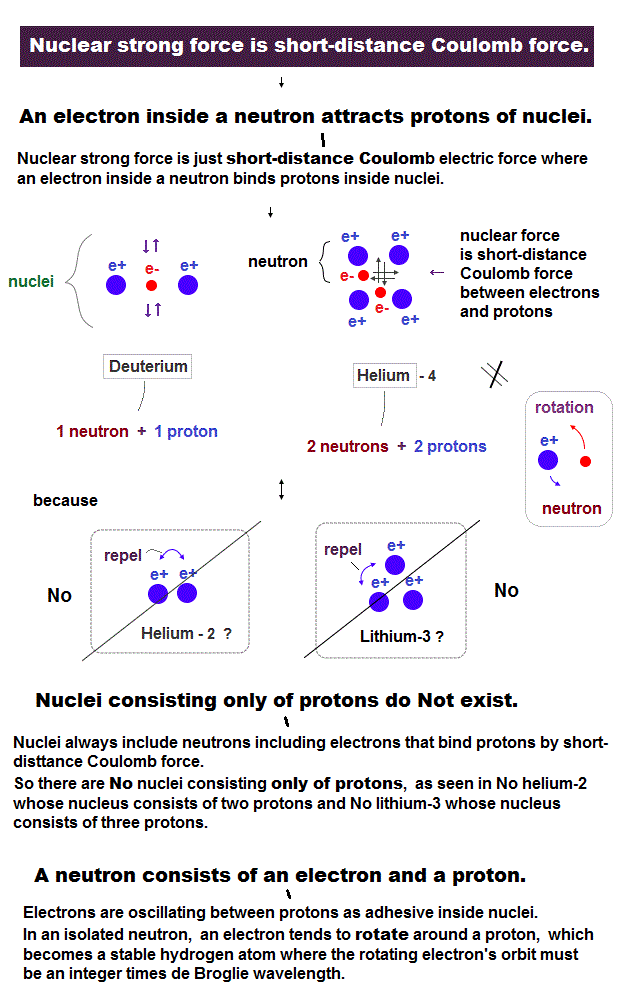Deuterium has one proton and one neutron.
So it is thought that an electron ( inside neutron ) attracts two protons between them.

In the same way, helium-4 having two protons and two neutrons uses two electrons as a "binder".  Nuclear space is very small, so each electron is quivering between protons.

On the other hand, in neutron, an electron tends to rotate around only one proton.
As I said, this orbital motion needs an integer times de Broglie wavelength, which breaks neutron.

More than one protons are indispensable for electron's oscillations among protons.
Like "spring" motion, this oscillation conserves energy and can be stable

## Why "negative" nucleus is impossible ?

### [ Proton is heavy and slow, it cannot "move around" to bind electrons ! ]

(Fig.10)  Heavy and slower protons cannot bind many electrons stably !Then why is each nucleus always positive, and it can never be negative ?
Eelectron is very light and moves much faster than heavy proton.

So each electron can capture protons, before slow protons go away from other protons.
But very heavy protons cannot play a role of nuclear "binder".

When there are more electrons than protons, each electron repelling each other, easily scatters.
Heavy proton cannot go ahead of electrons to prevent them from scattering.

This is the reason why each nucleus is always positive including neutrons (= electrons ).

## Quantum gravity (= 10 dimensional string ) is unreal !

### [ The only unified theory including "gravity" needs fantasy 10 spacetime dimensions ! ]

(Fig.11)  Graviton can cause "time dilation" ?  What mechanism ?The present physics is "disaster". There is only 10 dimensional string theory as unified theory with gravity.   So unless you believe extradimensions and 10500 many worlds, you will be persecuted.

These quantum gravity theories just show abstract "graviton" operators ( see this p.3 ), without ability to describe concrete physical mechanism.

They insist gravity causes time dilation. But how can each "graviton" handle the clock time, like magic ?  These are just unrealitic and strange.  Graviton by extradimensions are just "imaginary" concepts !

## Gravity is "macroscopic" effect of other fundamental forces.

### [ Very weak gravity is caused by low and high pressure fields' "tangle". ]

(Fig.12)  Field "imbalance" and their oscillation cause macroscopic gravity.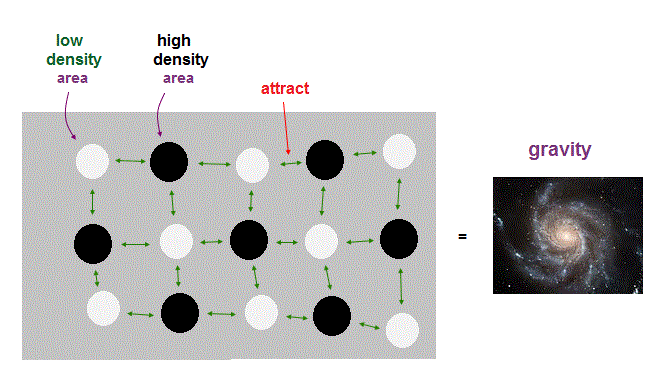It is known that gravity is extremely weaker (= about 10-39 times Coulomb force ! ) than other fundamental forces.  So it is natural to think gravity is NOT fundamental force but some macroscopic effect of other forces.

On this page, we can explain the Coulomb mechanism using "field density" imbalance.
Basically, "field" tends to be uniform in pressure, so high and low pressure regions attract each other.

We can think gravity as large-scale imbalance in field's density (= medium filling all space ).  If there are higher (= denser ) pressure areas, they tend to approach lower pressure areas.

When a large number of the same kind of field attraction synchronize, it generates "gravity", as macroscopic effect.  This idea is more natural than other "fantasy" 10 dimensions and graviton.

## Heavy proton is almost "at rest" in hydrogen atom.

(Fig.13)  Hydrogen atom.  Proton (= nucleus) is 1840 times heavier than electron.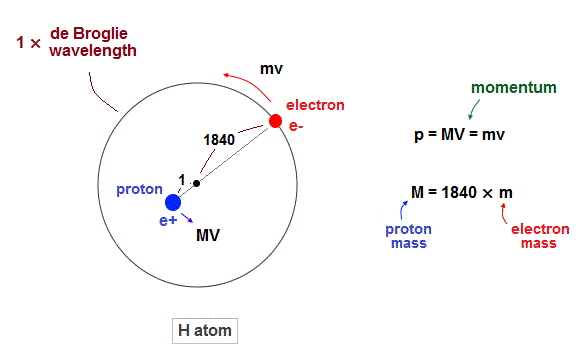Proton is about 1840 times heavier than an electron.
So proton (= nucleus ) is almost at rest in hydrogen atom.

But of course, from the fact of reduced mass, proton is thought to be moving by Coulomb force inside atoms.  Due to total momentum conservation, proton's momentum is always just the opposite of electron ( MV = mv ).

According to de Broglie theory ( wavelength = h/momentum ), both electron and proton's de Broglie wavelength should be the same. But they are not.

As seen in Fig.13, proton is confined to about 1840 times smaller region than the electron's orbit. So proton is almost at rest.

## Proton is almost stationary (= oscillation ) in small area.

(Fig.14)  While electron is orbiting, proton is almost "stationary".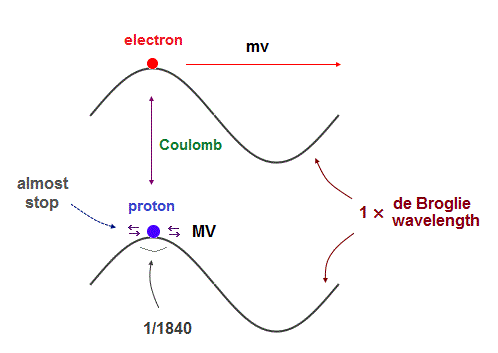Their same (= opposite ) momentums should generate the same de Broglie wavelength.
But proton's moving area is 1840 times shorter than electron's orbit.

So the proton is considered to be oscillating in almost the same place, instead of orbiting, like a electron inside neutron.

In fact, proton's magnetic moment (= g-factor × angular momentum ) is NOT proton's Bohr magneton, which is so weak that proton's actual "spinning" can influence its magnetism.

On the other hand, large magnetic moment of electron (= 658 times proton ) indicates it is due to electron's orbital motion, NOT by unrealistic tiny spin.

As light electron has to move to the opposite side of nucleus in which electron's uniform oscillation cannot be kept. So an electron must rotate around with de Broglie waves, not oscillate.

## Unrealistic positron does NOT satisfy energy conservation !

### [ 2 × rest mass energy = electron + positoron + "Coulomb energy" ? ← NOT conserved ! ]

(Fig.15)  After static electron, positron are created, Coulomb energy is -infinity !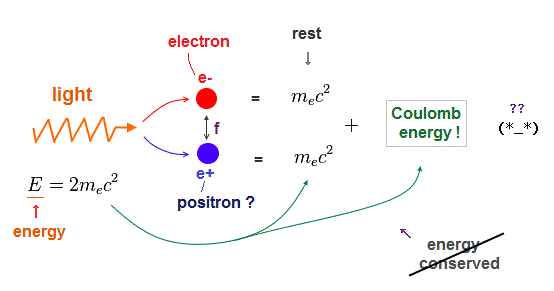It is known that antiparticles such as positrons do NOT obey total momentum conservation.  In fact, these antiparticles disobey energy conservation law, too !

In pair production, they say, when a photon has more energy than two rest mass energies ( mc2 of electron ), it can generate a pair of an electron and a positron.

But an electron and a positron has the oppoosite ± charges.  So the instant both these "static" particles are generated, they start to attract each other by Coulomb force.

This is strange. The first photon (= light ) energy is just 2mc2.  But just after an electron and proton (= at rest ) are created, their total energy becomes 2mc2 minus Coulomb potential !  Energy is NOT conserved !

(Fig.16)  Total energy is NOT conserved in pair production !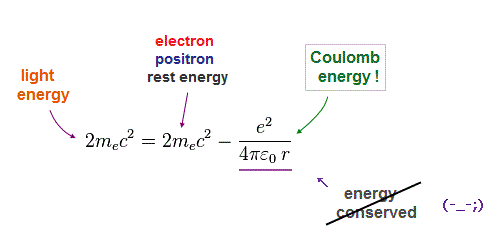This strong Coulomb attraction between stationary electron and positron show the total energy is NOT conserved in pair production.  So positron cannot prove Einstein mass relation ( E = mc2 ) at all !

In fact, antiparticles such as positrons cannot be isolated from other abundant electrons and protons.  So they just mistake electrons ( or protons ) scattered ( by Coulomb ) as antiparticles.

Because inside infinite different particles, we cannot know every single Coulomb scattering correctly.  It is impossible to determine vague antiparticle only from Lorentz magnetic force.

In this p.6,10, they say even after several different detectors, they may mistake proton for positron.  Of course these "mistake" probability itself is just speculation.  No body knows the exact effects of multi-particle scattering.

## Unreal Spin is too weak to explain ferromagnetism !

### [ Spin-Spin magnetic energy is too weak to cause ferromagnets at room temperature ! ]

(Fig.17)   Spin energy (= 1 K ) vs. actual ferromagnetic energy (= 1043 K ).Though ferromagnetism ( ex. permanent magnet of iron ) is said to be caused by spin ( see this ), this is impossible. Because spin-spin magnetic energy is too weak to explain this ferromagnet.

As shown in this p.6 , this p.1, this (= intro ), spin-spin dipole magnetic energy is only 0.0001 eV (= 1 K in temperature expression ), which is much lower than iron ferromagnetic temperature (= 1043 K ! ).

So they argue this ferromagnetism is caused by "quantum exchange" interaction (= Pauli ).
But quantum mechanics NEVER tries to clarify what this exchange force really is.

To express this strange exchange force, all they can do is rely on abstract math symbols.
In Hubbard spin ( see this p.5 ), it just shows meaningless electron operators, without ability to describe concrete atomic states, useless, forever.

## Ferromagnet is caused by strong "Coulomb" force !

### [ Atomic radius of Iron (= Fe ) causes stable rotation with neighboring atoms. ]

(Fig.18)  Spin is too weak !  Coulomb interaction causes ferromagnet !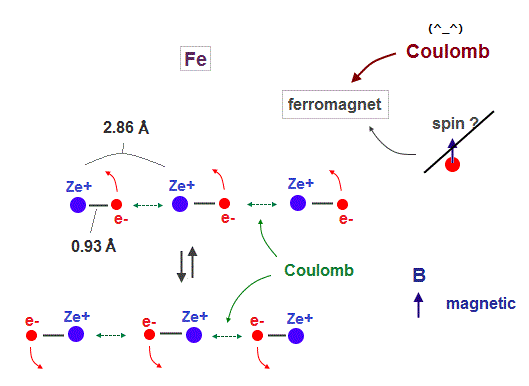It is known that iron (= Fe ), Ni, Co can be ferromagnet even at room temperature.
As I said, spin magnetic moment (= Bohr magneton ) is too weak (= 1 K ) to cause ferromagnet at 1043 K in Fe.

So we have to consider other strong interactions such as Coulomb forces.
In Fig.18, iron atomic raius (= 0.93 Å ) is suited for synchonizing with neighboring atoms.

Like in this section, if we designate de Broglie wavelength ( Fe = 4 ), atomic structure ( Fe = regular hexahedron ), and total 1-8th ionization energies, we can know the atomic radius ( Fe = 0.93 Å ). See this.

We use lattice constant of iron, which direction is most involved in ferromagnet.

## Titanium's atomic radius is "too large" for ferromagnet.

(Fig.19)  Titanium electron is too influenced by other neuclei.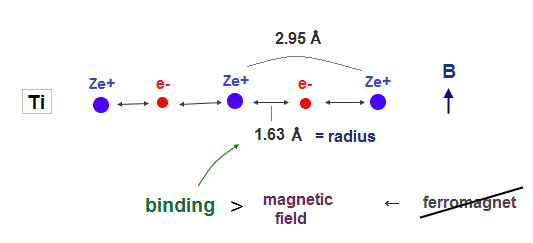In atoms such as titanium, which electron's number is smaller than iron, their atomic radius is longer due to weak central charge Ze+.

In this case, each valence electron is too much restricted by neighboring nuclei, which prevents them from responding to external magnetic fields.  As a result, ferromagnet cannot be seen in this titanium.

## Copper atomic radius "is too short" to synchronize with other nuclei.

(Fig.20)  Copper has 11 valence electrons. ← Too shrinked !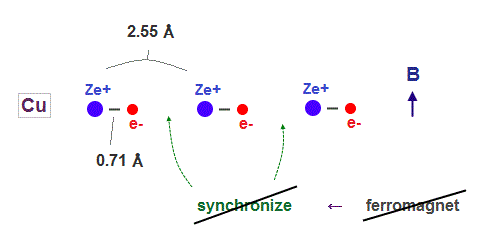On the other hand, copper has as many as 11 valence electrons.
So its atomic radius shrinks too much to sinchronize with other nuclei under external magnetic field.

In Copper, each electron is more influenced by other valence electrons belonging to the same atom, which prevents them from synchronizing with external magnetic fields.

As a result, Fe, Ni, Co have atomic radius, which length is just suited to cause stable ferromagnet.

## Quantized magnetic flux by de Broglie waves.

### [ Magnetic flux (= Φ ) inside a hole of superconductor is n × h/2e. ← de Broglie wave ! ]

(Fig.21)  Magnetic flux (= Φ ) inside hole is quantized = n × h/2e.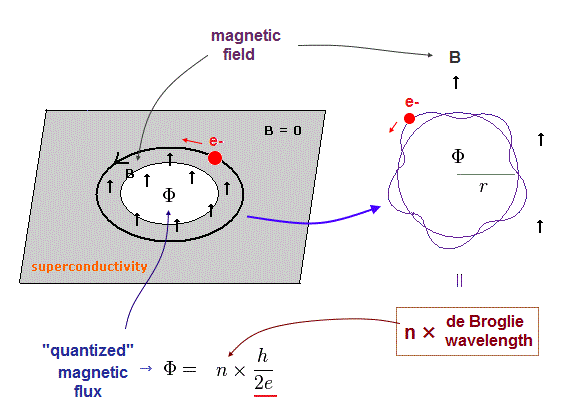It is known that magnetic flux (= Φ = B × area ) passing through (= trapped by ) the hole inside superconductor become quantized ( Φ = n × h/2e ).

Quantum mechanics tries to explain this quantized magnetic flux using Cooper with fictitious phonons and monopole.

But we can explain this phenomenon using real de Broglie waves.  See this p.23, this.

(Fig.22)  Lorentz force = centrifugal force.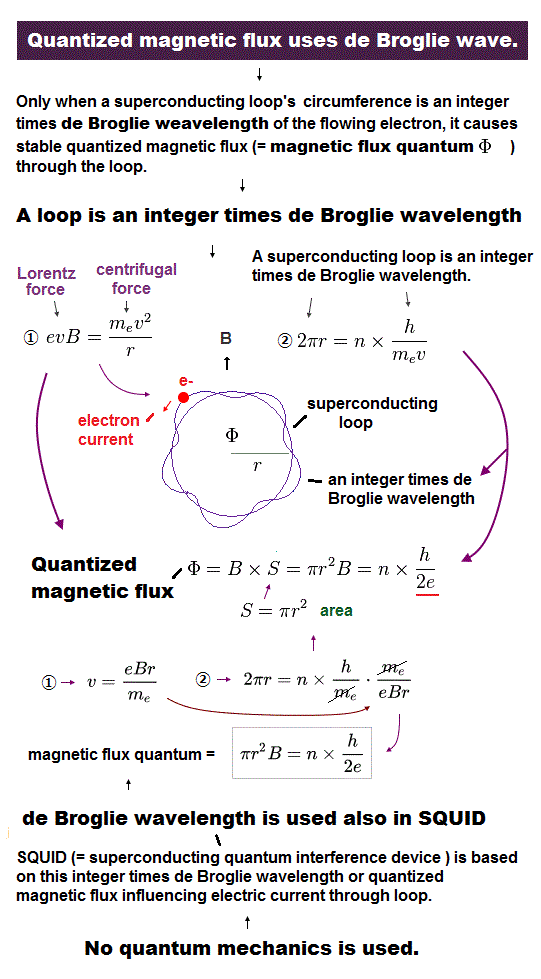When the applied magnetic field is B, Lorentz magnetic force becomes evB, which is perpendicular to electron's motion.  And the centrifugal force is mv2/r, where v and r are velocity and radius.

We assume the orbital length is just an integer (= n ) times de Broglie wavelength ( = h/mv ).  "h" and "m" are Planck constant and electron's mass.

(Fig.23)Solving two equations of Eq.22, we have Eq.23 and Eq.24.

(Fig.24)  Quantized magnetic flux (= Φ = n × h/2e ).The area of circle is "πr2", so magnetic flux (= Φ ) becomes B (= magnetic field ) × πr2, which result gives just an integer times magnetic flux quantum (= h/2e ) !

(Fig.25)So we can prove magnetic flux quntum using real de Broglie waves, NOT depending on "fictitious" quasiparticle phonon.

## Fracional quantum Hall effect by real de Broglie waves !

### [ Quantum mechanics aims only at "unreal" many-worlds, quasiparticles. ]

(Fig.26)   Quantum mechanics cannot solve many-body atoms → quasiparticle !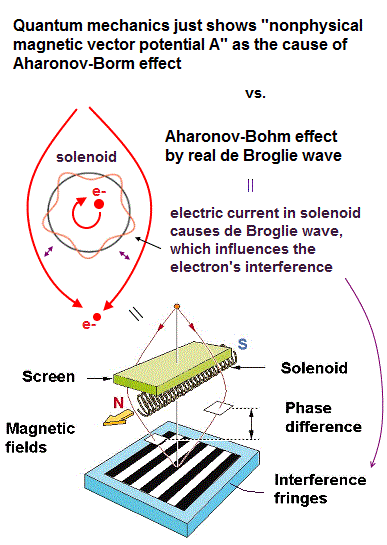According to phys.org, even recent journals (= Phys.Rev., PNAS ) deal with unreal anyon with fractional charge, though fractional charge ( ex. 1/3e ) cannot be isolated.

As shown in this, this, it's impossible to isolate and observe quarks with fractional charge in actual experiments.  So these anyons with fractional charge are just fictitious quasiparticles.

Quantum mechanics cannot solve and predict multi-electron atoms, and lacks reality in wavefunctions ( see many-worlds ), causing "camouflage" target, quasiparticle.

Quasiparticle are fictitious (= unreal ) elementary particles ( see this, this ).
But all the current condensed matter physics can do is invent these unreal particles.

Of course, we cannot directly observe fractional charges themselves.
They just artificially defined fractional charge only from fractional quantum Hall effect.

From the fact that electrical conductance can be a fraction × e2/h, they imagine fictitious anyons with fractional charge, which is just abstract math symbol with NO shape.  See this (1.1).

## Amount of electric current J is quantized by de Broglie waves !

### [ When external magnetic and electric fields are applied, its conductance is quantized. ]

(Fig.27)  Electric current J becomes "fraction" ×e2h E by de Broglie waves !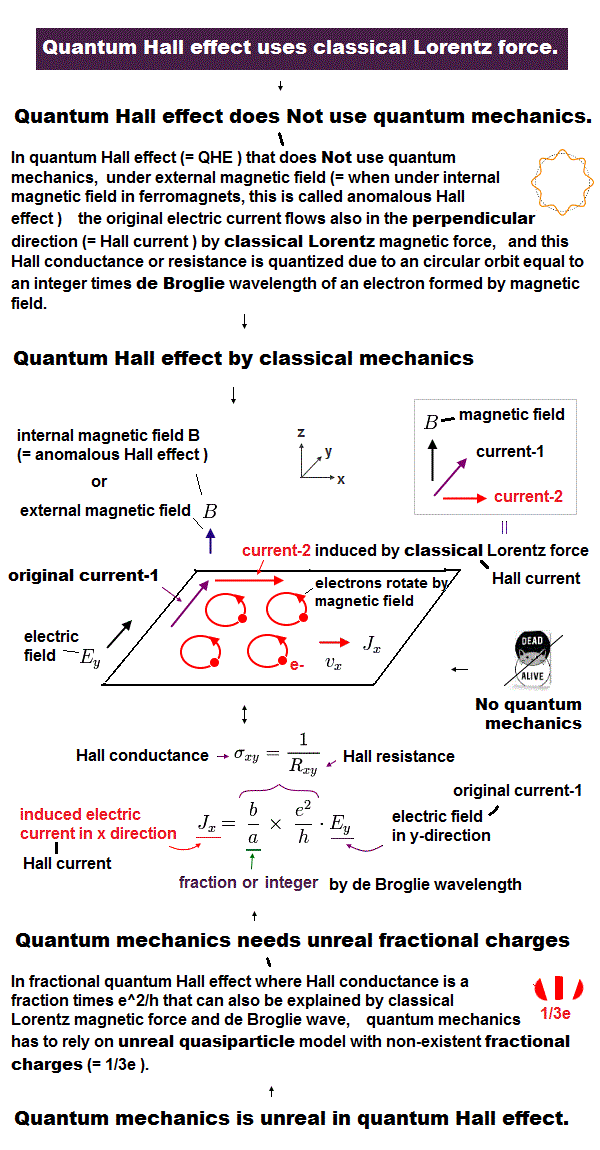In Fig.27, the electric field (= Ey ) is applied in y direction, and the magnetic field (= B ) is applied in z direction.  Each electron is rotating by Lorentz force.

Furthermore, this whole circular orbit is moving at velocity "v" in x direction.
Because applied electric force (= eEy ) and magnetic force (= evB ) are equal to each other.

(Eq.28)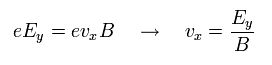From Eq.27, we find the balanced velocity in x direcction, as shown in Eq.28.

We assume each circular orbit satisfies an integer times de Broglie wavelength under mgnetic field B.

(Fig.29)  Each circular orbit in Fig.28 satisfies n × de Broglie wavelength !Solving Eq.29, the area (= πr2 ) of each circle becomes

(Eq.30)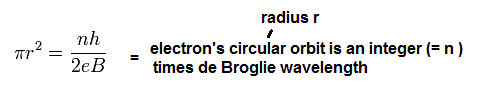The electric current (= J ) is equal to charge e × electron density (= N ) × velocity v.
Electron density N is equal to "k" (= number of electron included in one circle ) / πr2.

(Fig.31)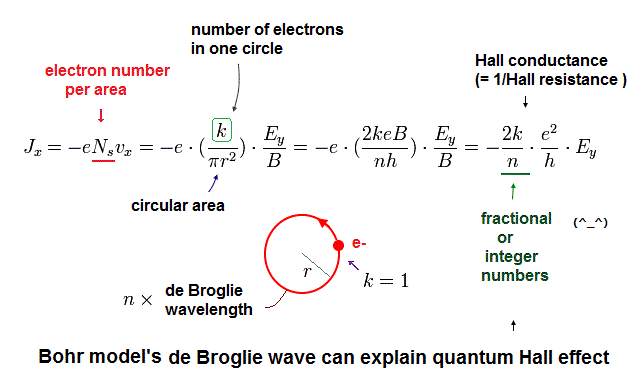From Eq.28, Eq.30, Eq.31, we obtain the value of fractional (= 2k/n ) quantum Hall effect.
"n" is de Broglie wavelength of each circular orbit. "k" is electron's number in one circle.

(Fig.32)  2 × de Broglie wavelength orbit.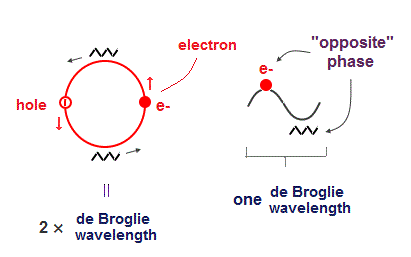Fig.32 shows 2 × de Broglie wavelength orbit.
This includes two pairs of the opposite charges.

We assume each orbit contains only one electron ( so k = 1, and another is "hole" )

## Each electron meets the "same" phases of other de Broglie waves !

(Fig.33)  Overlapping with the same phases in de Broglie waves makes them stable.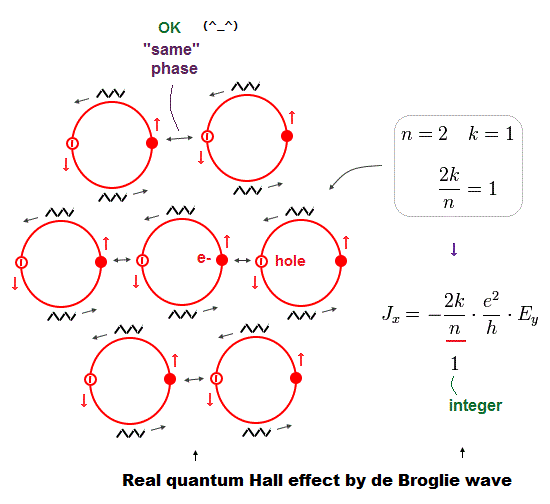As shown in Fig.33, an electron of each circular orbit always meets the same phase of other de Broglie waves.

The same-phase overlapping between each electron and hole makes each electron's circular motion stable.  In Fig.33, n = 2 (= de Broglie ) and k = 1 (= electron's number ), so an integer Hall effect.

## Each de Broglie wave avoids destructive interference !

(Fig.34)  Each electron always meets the same-phase hole !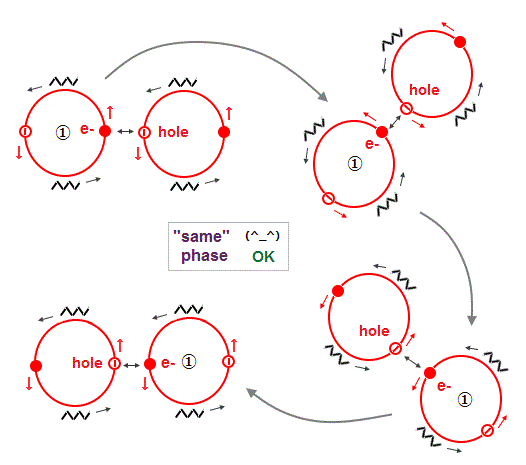As shown in Fig.33 and Fig.34, each electron always meets the same-phase hole of other de Broglie waves, avoiding destructive interference.

As a result, this electron's orbit and an integer ( 2k/n = 1 ) Hall conductance are stable with respect to de Broglie wave's interference.

## Multi-layer current causes an integer quantum Hall effect.

(Fig.35)  3 layers of electric currents gives "3" integer Hall effect !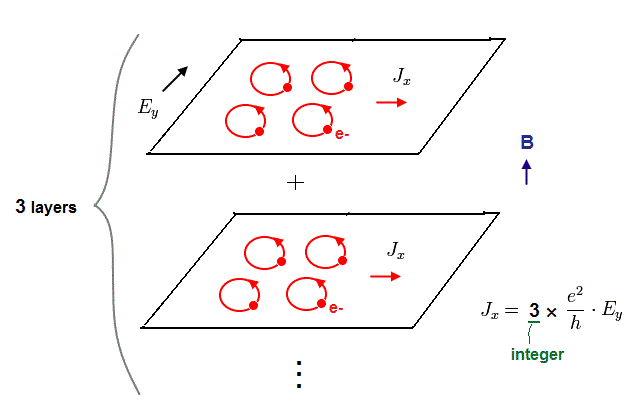Each layer of Eq.33 gives an integer "1" as the amount of electric current J in quantum Hall effect.
So when there are three layes, this integer becomes "3".

As a result, we can explain all integer quantum Hall effect !

## Spotted "empty" circular orbits → Fractional Hall effect !

(Fig.36)  A half of orbits are "empty" → fractional (= 1/2 ) quantum Hall effect.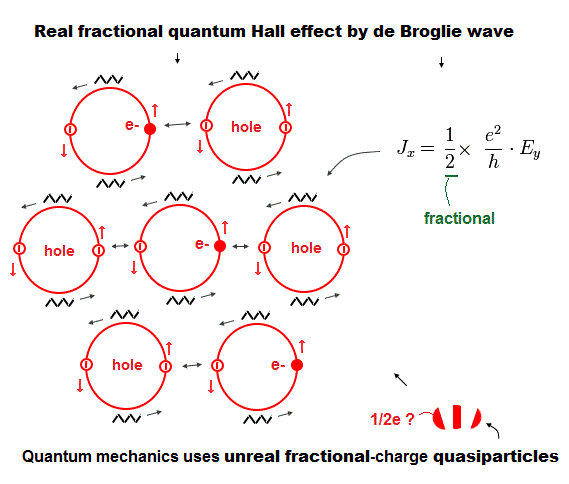When we reduce the whole amount of electric current, a part of circular orbits become empty with NO electrons.
Due to electric uniformity, the interval between empty orbits becomes equal.

In Fig.36, a half of circular orbits contains NO electrons.
In this case, "k" = 1/2, so it gives fractional (= 1/2 ) quantum Hall effect !.

If we think various different patterns using Fig.35 and Fig.36, we can explain all kinds of fractional (+ integer ) quantum Hall effect, NOT relying on "fantasy" anyon.

## de Broglie "wavelength" determines the maximum orbits.

(Fig.37)  Maximum orbits = midpoint lines + 2 (= two perpendicular orbits )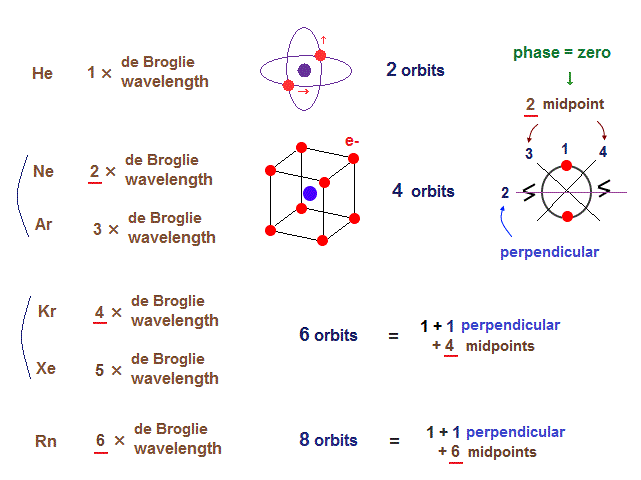On this page, we show all atomic structures and the number of midpoint lines ( related to de Broglie wavelength ) influences the number of maximum orbital number in the periodic table.

In this section, we prove atomic structure based on de Broglie wavelength can explain various molecular bond length.

## True radius of helium-like atoms

### [ An integer times de Broglie wavelength gives true atoic radius. ]

(Fig.38)  Rough estimtion of helium-like structure.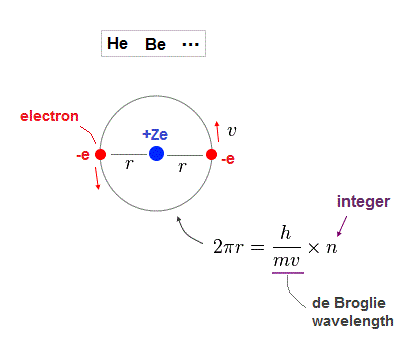As shown on this page, when two electrons in the same orbit, it gives a little different energy values.
( Two perpendicular orbits give the exact helium ground state energy. ).

But approximately, Fig.38 simple helium-like model can be used in estimating atomic radius.
One orbital length is supposed to be an integer times de Broglie wavelength in Fig.38.

Nuclear central charge is +Ze, atomic radius is "r".  "v" is electron's velocity

(Fig.39)  Coulomb force = centrifugal force.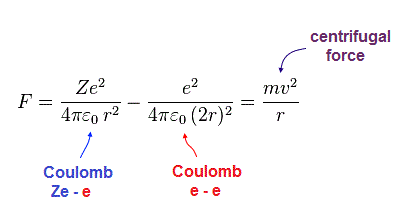In Fig.39, the sum of Coulomb force ( from nucleus and another electron ) is equal to the centrifugal force.

(Fig.40)  Total energy E = sum of potential and kinetic energies.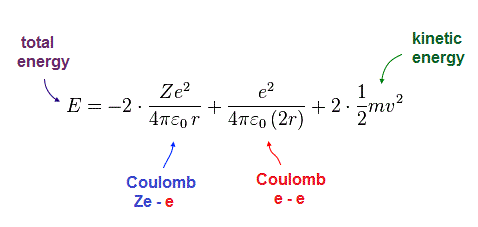Total energy E is the sum of all potential and kinetic energies.
Solving Fig.38-40, we can obtain the equations of atomic radius "r" and total energy "E".

(Fig.41)  Atomic radius "r" and total energy E depend on de Broglie wavelength "n".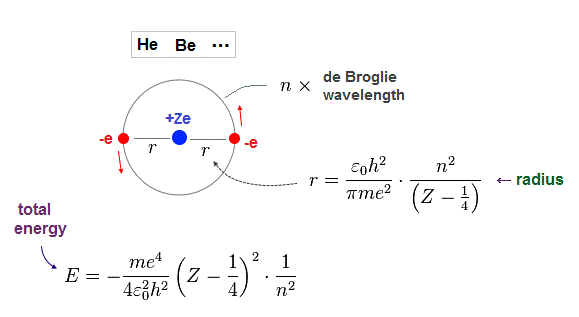Just for calculation, we use Bohr radius and ground state energy of hydrogen atom.

(Fig.42)  Hydrogen Bohr radius and ground state energy.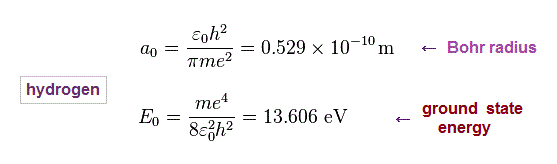Beryllium has two valence electrons in n = 2 orbits.
We suppose the sum of 1st and 2nd ionization energies of Be is total energy E in n = 2 orbits.

Inserting E = 27.5 eV (= sum of 1st, 2nd ionization energies ) and n = 2 (= de Broglie wavelength ) into the result of Fig.41, we obtain Fig.43.

## When 2 × de Broglie wavelength, it gives correct central charge.

(Fig.43)  Beryllium effective central charge Z = 2.26. ← good !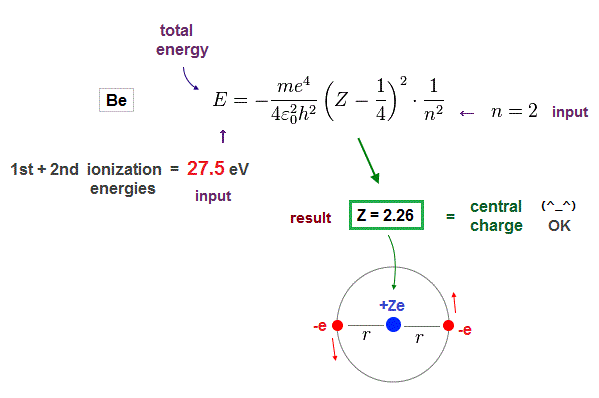As seen in Fig.43, when we input n = 2 as de Broglie wavelength, it gives reasonable effective central charge Z = 2.26.

This central charge is the sum of +4e Be nucleus and two 1s electrons. ( +4e -2e = +2e )
So the sum of them should be close to "2".  Due to electron 1s shell gap, it's a little bigger than 2.

(Fig.44)  Atomic radius when 2 × de Broglie wavelength in Be.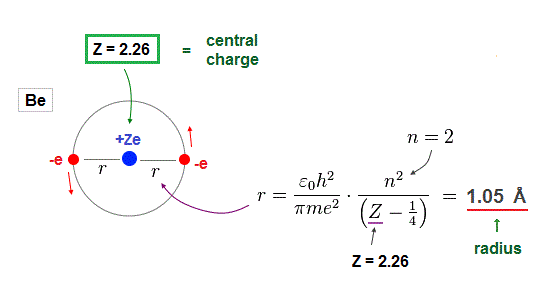Furthermore, when Be valence electrons are in 2 × de Broglie wavelength orbits, their orbital radius becomes 1.05 Å, which can explain experimental bond length properly.

## Atomic radius by de Broglie wavelength explains exact bond length !

(Fig.45)  Experimental Be-Be bond length = 2.22 Å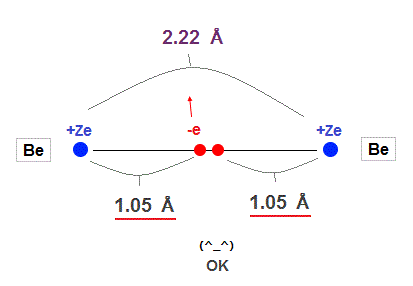In this site, the experimental value of Be-Be bond length is 2.22 Å ( 1 Å = 1.0 × 10-10 m ).
This value is almost 2.10 Å = 2 × 1.05 Å of calculation value in Fig.44 !

So the concept of de Broglie wavelength is valid in Beryllium molecular bond !

## When Be valence electron is in 1 × de Broglie wavelength ..

### [ If Be valence electrons are in 1 × de Broglie wavelength, it gives wrong results ! ]

(Fig.46)  n = 1 → Central charge Z is too small, and radius is too short !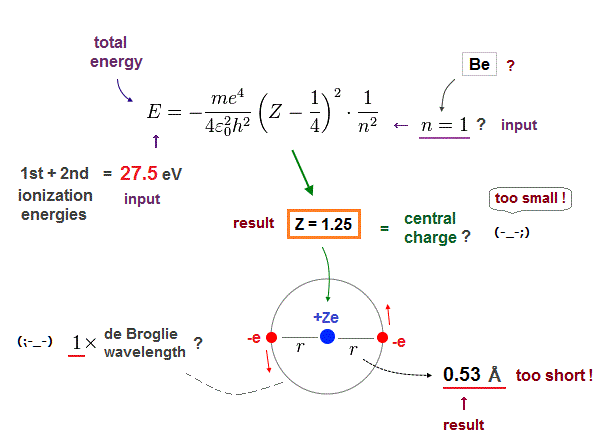Next we suppose Beryllium valence electrons are in 1 × de Broglie wavelength orbits and input n = 1 instead of n = 2.  The same ionization energy (= 27.5 eV ) is used as total energy E.

As shown in Fig.46, in this case, the effective central charge becomes too small ( Z = 1.25 ).
Central charge must be bigger than 2, so this result is inconsistent with the actual Beryllium !

Orbital radius is also too short (= 0.53 Å ).
2 × 0.53 Å = 1.06 Å is much shorter than the actual bond length ( 2.22 Å ).

## When Be valence electron is in 3 × de Broglie wavelength ..

### [ If Be valence electrons are in 3 × de Broglie wavelength, it also gives wrong results ! ]

(Fig.47)  Central charge Z is too big, and radius r is too short in n = 3 !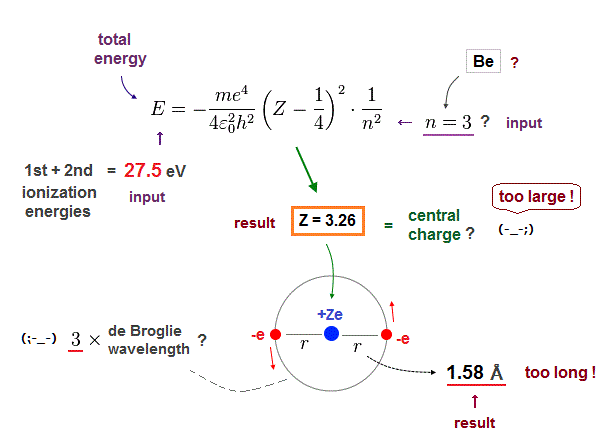Next, if we input n = 3 instead of n = 2 into these equations.
This case also gives unreasonable results.

The effective central charge Z = 3.26 is too big, with respective to "2".
And atomic radius 1.58 Å is too long to explain actual Be-Be bond length (= 2.22 Å ).

As a result, we can prove two valence elecrons of Beryllium in 2 × de Broglie wavelength orbit !

## Carbon-like atomic radius by de Broglie wavelength.

(Fig.48)  Carbon = four valence electrons.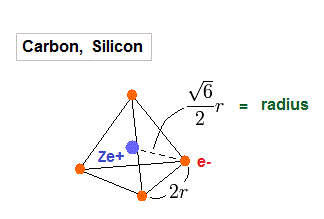Carbos and silicons have four valence electrons.
So we assume regular tetrahedron like Fig.48 to get atomic radius.

(Fig.49)  Total potentian energy V.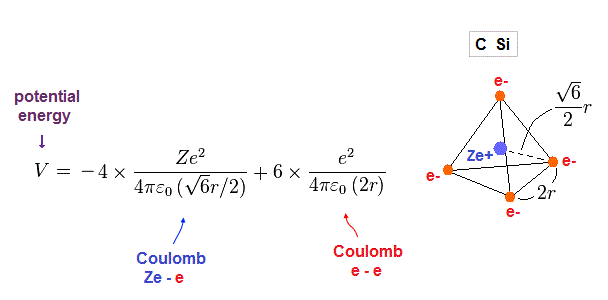Total potential energy is the sum of energies among Ze+ center and four electrons.

(Fig.50)  Coulomb force toward center acting on each electron.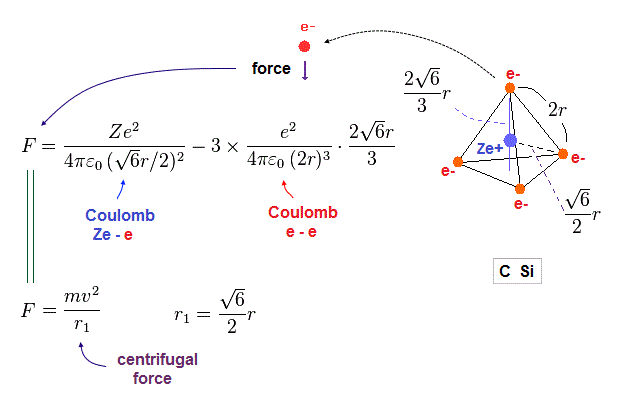Fig.50 is the Coulomb force toward center acting on each electron.
We suppose this force is equal to centrifugal force.

(Fig.51)  n × de Broglie wavelength orbit !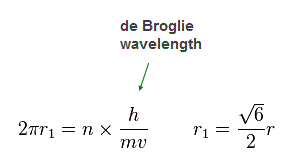Again we suppose each orbit satisfies an integer (= n ) times de Broglie wavelength.

(Fig.52)  Total energy E and radius r in carbon-like atoms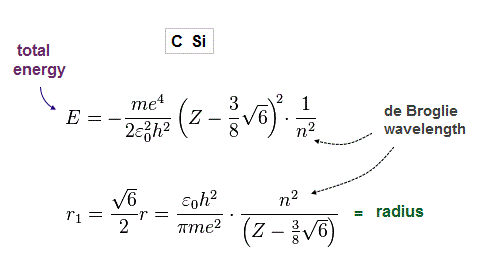From Fig.49-51, we obtain total energy E and radius r, as shown in Fig.52.

## 2 × de Broglie wavelength gives right values in carbon !

(Fig.53)  True atomic radius r and effective central charge Z in Carbon.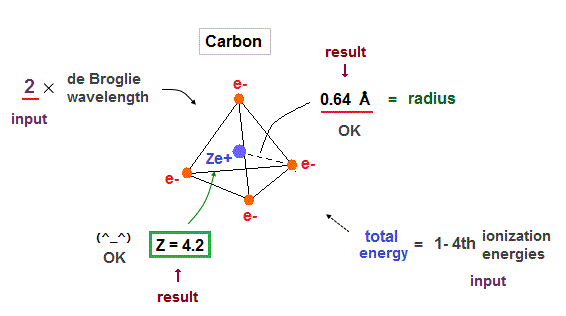In this table, we suppose the sum of 1-4th ioniztion energies as total energy of n = 2 carbon valence electrons.

Inputting E = 148 eV and n = 2 into Fig.52, we get radius 0.64 Å and Z = 4.2, which is very good.

## Molecular bond length can be explained by de Broglie waves !

(Fig.54)  Experimental values of C-H, C-C bond length.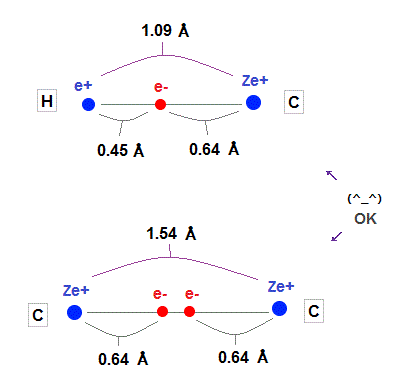As shown on this and this, experimental values of H-C and C-C bond length is 1.09 Å and 1.54 Å
Carbon atomic radius (= 0.64 Å ) fits these experimental values very well.

Hydrogen atomic radius (= 0.45 Å ) shrinks, and is a little shorter than Bohr radius (= 0.53 Å ) due to attraction of carbon's nucleus.

## 1 or 3 de Broglie wavelength gives wrong results in carbon !

(Fig.55)  If carbon valence electrons are in 1 or 3 de Broglie wavelength, wrong radius !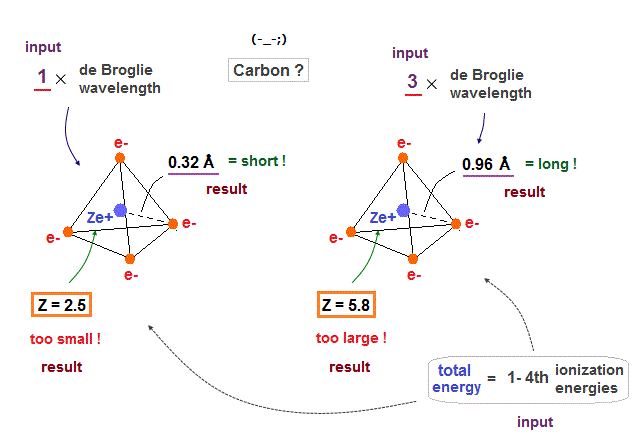If we suppose carbon's valence electrons are in 1 or 3 de Broglie wavelength orbits, it gives "too short" or "too long" atomic radius, compared with the experimental bond lenghs.

Effective central charge (= Z ) are also "too small" (= 2.5 ) or "too large" (= 5.8 ) compared with the proper value of "4".  So we find four valence electrons of carbon are in 2 × de Broglie wavelength orbits.

## Silicon atomic radius based on de Broglie wavelength = 3.

(Fig.56)  Silicon radius and central charge, when 3 × de Broglie wavelength.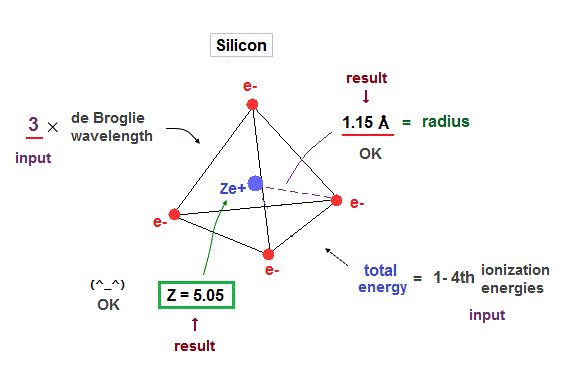When we suppose four valence electrons of silicon are in 3 × de Broglie wavelength orbits, it gives good results.  Effective central charge (= 5.05 ) is a little bigger than carbon, due to larger gap.

## 2 or 4 de Broglie wavelengths give wrong results in silicon !

(Fig.57)  When silicon valence electrons are in 2 or 4 de Broglie wavelength orbits ..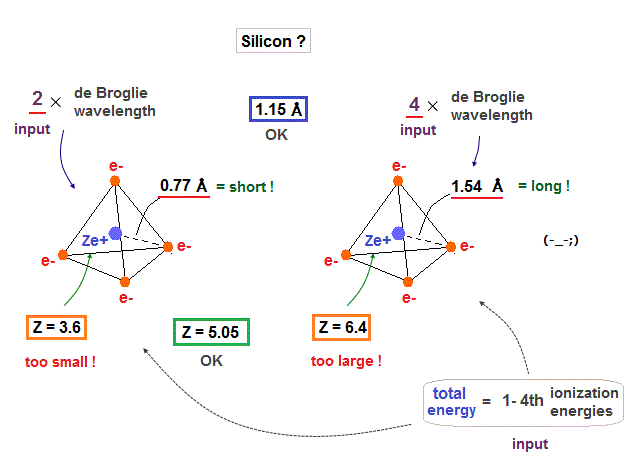If we suppose silicon valence electrons are in 2 or 4 de Broglie wavelength orbits, their effective charges become "too small" ( Z = 3.6 ) or "too big" ( Z = 6.4 ), compared with 4.

## 3 × de Broglie wavelength explain experimental bond length in silicon !

(Fig.58)  Experimental value of Si-Si bond length.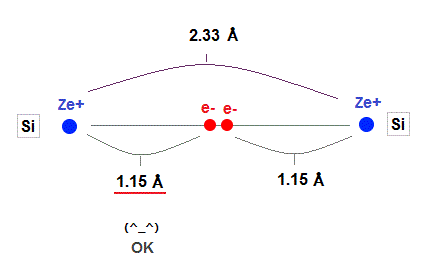As shown in this, the exeprimental value of Si-Si bond length is 2.33 Å.
Atomic radius in 3 × de Broglie wavelength is just consistent with this experimental bond length.

1.15 × 2 = 2.30 Å = 2.33 Å, when 3 × de Broglie wavelength !
On the other hand, radii in 2 or 4 de Broglie wavelength are inconsistent with the experimental values !

## Larger inner shell "gap" gives larger effective charges.

(Fig.59)  Why silicon's effective central charge (= 5.05 ) is bigger than carbon (= 4.2 ) ?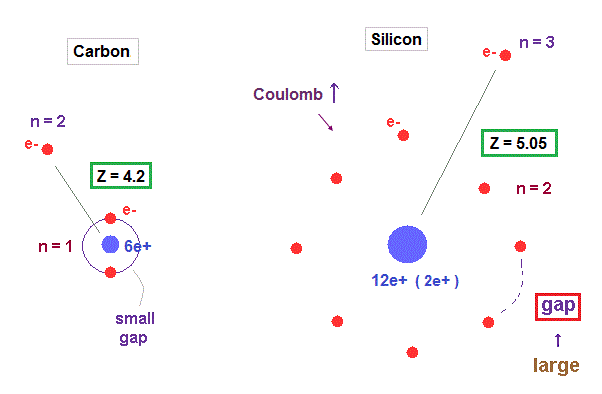Though both carbon and silicon have the same four valence electrons, their effective central charges (= Z ) are different.  Because larger gaps are open in silicon inner shell.

As shown in Fig.59, gap between n = 1 electrons are much smaller than that of n = 2 electron shell, which difference gives larger effective central charge Z in silicon.

## Oxygen-like atomic radius by de Broglie waves.

(Fig.60)  Regular octahedron = Oxygen-like.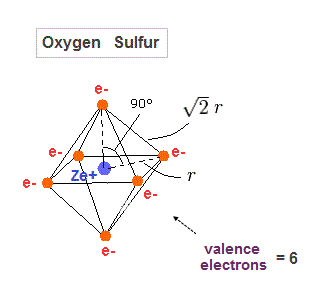Oxygen and sulfur have six valence electrons, so we can suppose they have "octahedral" electron distribution.

(Fig.61)  Total potential energy V in oxygen-like atoms.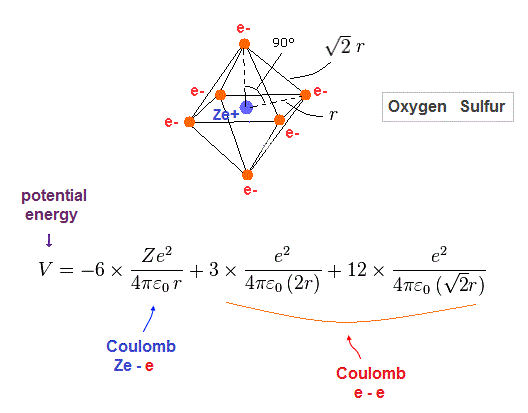Fig.61 is total potential energy V in oxygen-like atoms.

(Fig.62)  Force acting on each electron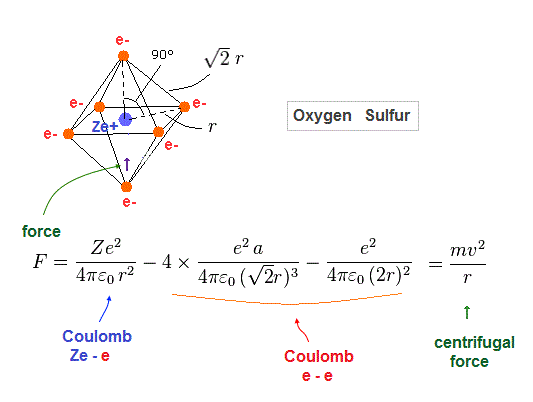Fig.62 is Coulomb force acting on each electron in oxygen-like atoms.
We suppose this is equal to centrifugal force.

(Fig.63)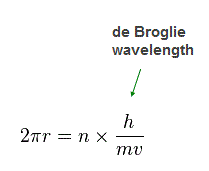Again we suppose each orbit is n × de Broglie wavelength.

(Fig.64)  Total energy E and radius of oxygen-like atoms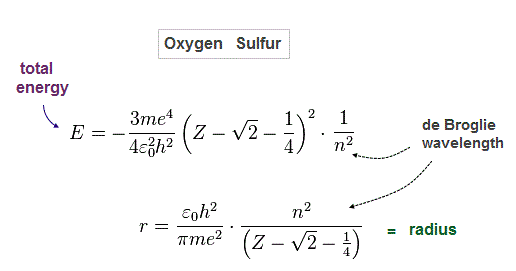Solving Fig.61-63, we get Fig.64.

(Fig.65)  Oxygen ( Sulfur ) is 2 (3) × de Broglie wavelength.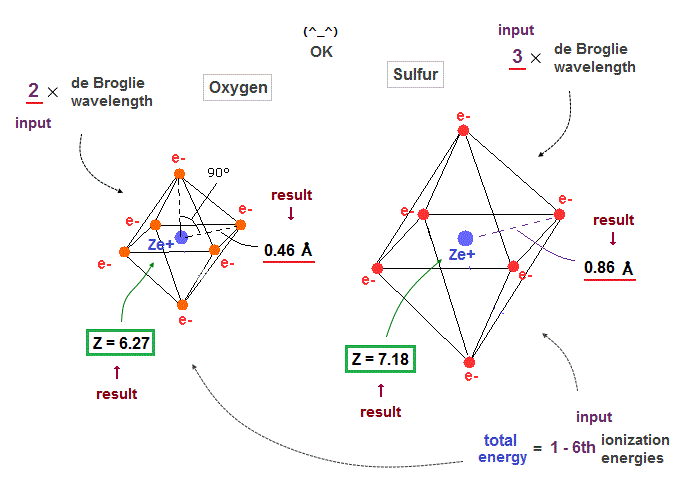When we suppose oxygen valence electrons are 2 × de Broglie wavelength orbits, it gives good central charge ( Z = 6.27 ), which is close to "6".

Due to larger gap in inner shell, the effective central charge Z of sulfur becomes a little larger (= 7.18 ) than oxygen.

## de Broglie waves can explain actual bond length in oxygen-like atoms !

(Fig.66)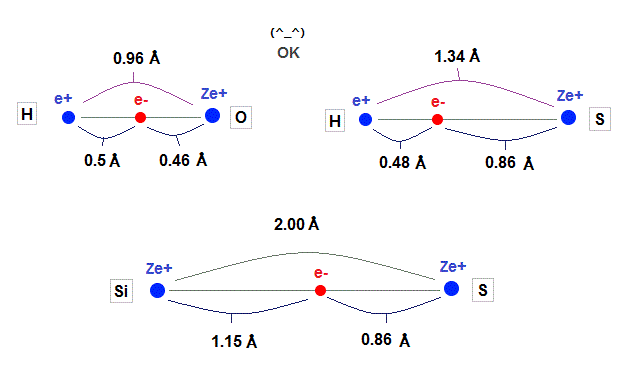Using calculation results by de Broglie wavelength in oxygen-like and silicon, we can explain experimental bond length, as shown in Fig.66.

## Coulomb interaction is a main factor in bond length.

### [ When interatomic distance is too short, Coulomb replusion gets stronger ! ]

(Fig.67)  When two atoms approach too close, repulsion among "electrons" get bigger.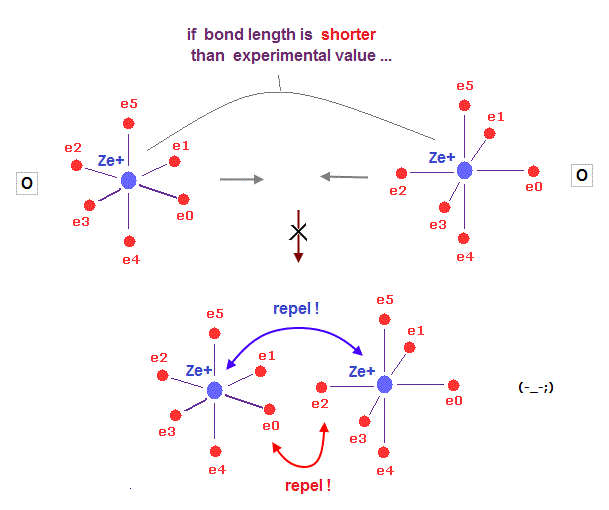What determines each molecular bond length ?

On this page, we prove Coulomb repulsion among electrons becomes stronger, when the distance between two atoms is shorter than the experimental value in H2, using Virial theorem.

As two nuclei are closer to each other, electrons in both atoms are attracted toward the center of two atoms. This repulsion among electrons gets stronger in shorter bond length.

## Why hydrogen H is attracted toward fluorine F so much ?

### [ A single electron in H atom can pass through small electron "gap" in fluorine. ]

(Fig.68)   H - F bond is very tight and stable. Why ?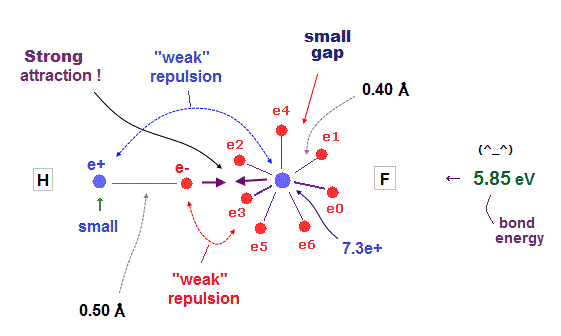It is known that hydrogen tends to be attracted and bound to fluorine tightly and stably.
Fluorine has seven valence electrons and large effective central charge, so its radius is very short.

So there are only very small gaps among seven valence electrons in F.
To avoid electrons and pass through this small gap, another atom must have a small number of valence electrons.

This is the reason why hydrogen atom with only one electron tends to be bound to fluorine tightly.

## Many electrons between fluorines repel each other.

(Fig.69)  F-F bond is very unstable due to strong repulsion among electrons.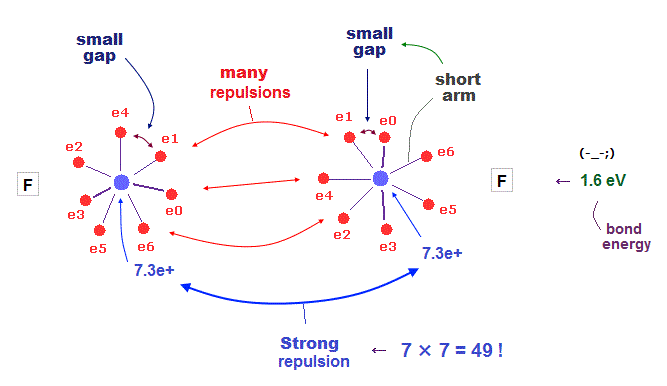When two fluorines are bound to each other, this F-F bond tends to be unstable.
Because both these two fluorines have very small electrons' gaps.

To cancel strong internuclear (= 7e × 7e ) repulsions, both-side electrons have to approach another nucleus.  But very small gaps among valence electrons in F prevent them.

This is the true mechanism of molecular bond length.
If you run this java program, you can know radius (= nuc ) and central charge (= Z ).

## Atomic radius and central charge by de Broglie waves.

Atom electrons de Broglie Radius charge Z
H 1 1 0.45 Å Z = 1.0
Be 2 2 1.05 Å Z = 2.2
C 4 2 0.64 Å Z = 4.2
N 5 2 0.53 Å Z = 5.2
O 6 2 0.46 Å Z = 6.3
F 7 2 0.40 Å Z = 7.3
Al 3 3 1.39 Å Z = 4.0
Si 4 3 1.15 Å Z = 5.0
P 5 3 0.98 Å Z = 6.1
S 6 3 0.86 Å Z = 7.2
Cl 7 3 0.76 Å Z = 8.2

## Atomic radius and central charge, Na - Zn.

Atom electrons de Broglie Radius charge Z
Na 1 3 2.59 Å Z = 1.8
Mg 2 3 1.74 Å Z = 2.9
K 1 4 3.74 Å Z = 2.2
Ca 2 4 2.60 Å Z = 3.5
Ti 4 4 1.63 Å Z = 6.1
Cr 6 4 1.18 Å Z = 8.8
Mn 7 4 1.04 Å Z = 10.2
Fe 8 4 0.93 Å Z = 11.5
Co 9 4 0.85 Å Z = 12.8
Ni 10 4 0.77 Å Z = 14.2
Cu 11 4 0.71 Å Z = 15.5
Zn 12 4 0.66 Å Z = 16.8

## Ionization energies of various atoms and ions.

(App.3) Ionization energies of atoms and ions (eV).
( Experimental values, CRC. )
Atom 1st 2nd 3rd 4th 5th 6th 7th Total
H 13.606 - - - - - -  13.606
Be 9.323 18.211 - - - - -  27.534
C 11.260 24.383 47.888 64.494 - - - 148.025
O 13.618 35.121 54.936 77.414 113.899 138.120 - 433.108
F 17.423 34.971 62.708 87.140 114.243 157.165 185.186 658.836
Si 8.152 16.346 33.49 45.142 - - -  103.13
P 10.487 19.77 30.202 51.444 65.025 - -  176.928
S 10.36 23.338 34.79 47.222 72.595 88.053 -  276.358
Cl 12.968 23.814 39.61 53.465 67.8 97.03 114.196  408.8832015/6/7 updated. Feel free to link to this site.